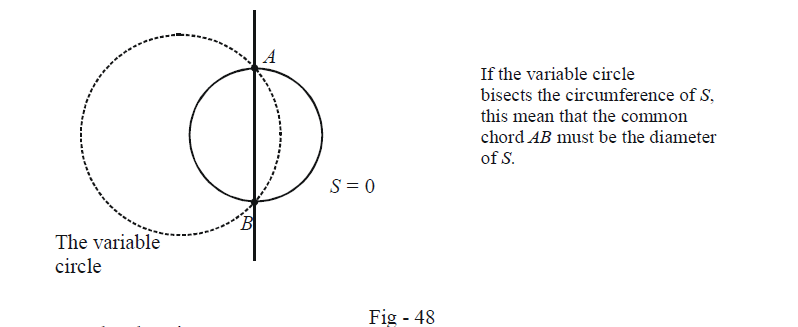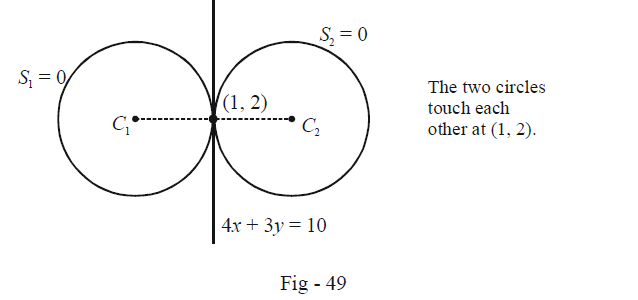# Examples On Family Of Circles

Go back to  'Circles'

Example - 38

A family of circles passing through the points A (3, 7) and B (6, 5) cuts the circle $${x^2} + {y^2} - 4x - 6y - 3 = 0.$$ Show that the common chord of the fixed circle and the variable circle(belonging to the family) will always pass through a fixed point. Find that point.

Solution: We can write the equation to the specified family of circles by first writing the equation L of the line AB:

\begin{align} L&:\frac{{y - 7}}{{x - 3}} = \frac{2}{{ - 3}}\\ \Rightarrow \quad L&:2x + 3y = 27\end{align}

The required family can now be written as

\begin{align}& F:(x-3)(x-6)+(y-7)(y-5)+\lambda L=0\;\text{ }\!\!~\!\!\text{ where }\lambda \text{ }\!\!~\!\!\text{ }\in \mathbb{R} \\ \Rightarrow \qquad & \text{ }\!\!~\!\!\text{ }F:{{x}^{2}}+{{y}^{2}}+(2\lambda \text{ }\!\!~\!\!\text{ }-9)x+(3\lambda \text{ }\!\!~\!\!\text{ }-12)y+(53-27\lambda )=0 \\ \end{align}

The common chord of F and the given circle S is:

\begin{align}&\qquad\;\; S - F = 0\\&\Rightarrow\quad (5 - 2\lambda )x + (6 - 3\lambda )y + (27\lambda - 56) = 0\\ &\Rightarrow \quad (5x + 6y - 56) - \lambda (2x + 3y - 27) = 0\\ &\Rightarrow \quad {L_1} + \mu {L_2} = 0\end{align}

That the common chord can be written like $${L_1} + \mu {L_2} = 0$$ implies that it will always pass through the intersection point of L1  and L2 , what ever the value of $$\mu$$ may be. This intersection point can be obtained (by simultaneously solving L1  and L2 ) to be \begin{align}\left( {2,\,\frac{{23}}{3}} \right)\end{align}.

Example - 39

Find the equation of the circle which touches the line $$x - y = 0$$ at the origin and bisects the circumference of the circle $${x^2} + {y^2} + 2y - 3 = 0$$ .

Solution: In example -36, we evaluated the equation of the family of circles all touching a given line at a given point. Here, the given line is $$x - y = 0$$ and the given point is (0, 0). Thus, the equation of the family is

\begin{align} & F:{(x - 0)^2} + {(y - 0)^2} + \lambda (x - y) = 0\\ \Rightarrow \quad & F:{x^2} + {y^2} + \lambda x - \lambda y = 0 \qquad \qquad \qquad \qquad ...(1)\end{align}

We need to find the value of $$\lambda$$ for which the circle in (1) bisects the circumference of the given circle $$S:{x^2} + {y^2} + 2y - 3 = 0,$$ which means that the common chord of the required circle and S will the diameter of S.The common chord AB is

\begin{align}&F - S = 0\\ \Rightarrow\qquad &\lambda x - (\lambda + 2)y + 3 = 0\end{align}

Since this is the diameter of S, the centre of S, i.e. (0, –1), must lie on it (satisfy its equation). Thus, we obtain $$\lambda$$ as

\begin{align} & \lambda (0) - (\lambda + 2)( - 1) + 3 = 0\\ \Rightarrow \quad & \lambda = - 5\end{align}

Finally, we substitute this value of $$\lambda$$ back in (1) to get the required equation of the circle as

${x^2} + {y^2} - 5x + 5y = 0.$

Example - 40

Two circles, each of radius 5 units, touch each other at (1, 2). If the equation of their common tangent is $$4x + 3y = 10,$$ find the equation of the circles.

Solution: The following diagram explains the situation better:We describe here two alternatives that can be used to solve this question

Alternative - 1: Find the centres of the two circles

The slope of the common tangent is \begin{align} - \frac{4}{3}.\end{align} Therefore, the slope of C1 C2  is  \begin{align}\frac{3}{4},\end{align} i.e.

\begin{align} & m = \tan \theta = \frac{3}{4}\\ \Rightarrow \qquad & \sin \theta = \frac{3}{5}\;{\text{and}}\;\cos \theta = \frac{4}{5} \end{align}

Using the polar form, we can write the co-ordinates of any point on the  line C1 C2 :

\begin{align}&\,\,\,\,\,\,\,\,\,\,\,\,\,\,\,\,\,\,\frac{{x - 1}}{{\cos \theta }} = \frac{{y - 2}}{{\sin \theta }} = r\\&\Rightarrow \qquad x = 1 + r\cos \theta ,\,\,\,y = 2 + r\sin \theta\end{align}

Substituting $$r = \pm \,5$$ gives the two centres as should be apparent from the  figure. Thus, the two centres are

${C_1} \equiv (5,\,\,5)\;{\rm{and}}\;{C_2} \equiv ( - 3,\,\, - 1)$

Thus, the two equations are

\begin{align}&{S_1}:{x^2} + {y^2} - 10x - 10y + 25 = 0\\&{S_2}:{x^2} + {y^2} + 6x + 2y - 15 = 0\end{align}

Alternative - 2: Use a family of circles approache

The family of circles touching L = 0 at $$({x_1},{y_1})$$ can be written, as described earlier, as

${(x - {x_1})^2} + {(y - {y_1})^2} + \lambda L = 0$

In the current case, this becomes

\begin{align} & \qquad\; {{(x-1)}^{2}}+{{(y-2)}^{2}}+\lambda (4x+3y-10)=0 \\ & \Rightarrow \quad {{x}^{2}}+{{y}^{2}}+(4\lambda -2)x+(3\lambda -4)y+(5-10\lambda )=0\quad \quad ......(1) \\ \end{align}

The radius of the required circle is 5. Thus,

${{(2\lambda -1)}^{2}}+{{\left( \frac{3\lambda -4}{2} \right)}^{2}}-(5-10\lambda )={{5}^{2}}$

This is a quadratic in $$\lambda$$  which gives two values of $$\lambda$$ :

$\lambda = \pm 2$

Using these values in (1), we obtained the two required circles.

## TRY YOURSELF - IV

Q. 1 Find the equations of the circles with radius 4 and passing through the points of intersection of

\begin{align}&{S_1}:{x^2} + {y^2} - 2x - 4y - 4 = 0\\&{S_2}:{x^2} + {y^2} - 10x - 12y + 40 = 0\end{align}

Q. 2 Find the equation of the circle whose diameter is the common chord of the circles $${(x - a)^2} + {y^2} = {a^2}$$  and $${x^2} + {(y - b)^2} = {b^2}$$ .

Q. 3 Find the equation of the circle passing through (2, 1) and touching the line $$x + 2y = 1$$ at (3, –1).

Q. 4 Tangents are drawn from the origin to $${x^2} + {y^2} + 6x + 4y - 12 = 0.$$ Find the equation of the circle passing through the points of contact of these tangents and the origin.

Q. 5 Find the equation of a circle which touches the line $$x + y = 5$$ at the points (–2, 7) and cuts the circle $${x^2} + {y^2} + 4x - 6y + 9 = 0$$ orthogonally.